# Practice Questions: Statistics Notes | Study Mathematics (Maths) Class 9 - Class 9

## Class 9: Practice Questions: Statistics Notes | Study Mathematics (Maths) Class 9 - Class 9

The document Practice Questions: Statistics Notes | Study Mathematics (Maths) Class 9 - Class 9 is a part of the Class 9 Course Mathematics (Maths) Class 9.
All you need of Class 9 at this link: Class 9

Question 1. Following are the marks obtained by 40 students of class IX in an examination: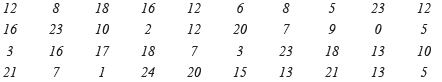(i) Present the data in the form of frequency distribution using the same class size such as 0–5, 5–10, etc.
(ii) How many students obtained marks below 15?
Solution:
The lowest value of the observation = 1
The highest value of the observation = 24
∴ To cover all the given data, the required classes are: 0–5, 5–10, …, 20–25.
(i) The required frequency distribution is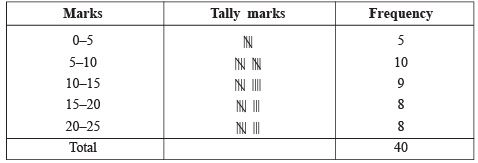(ii) From the table, it is found that number of students who have obtained marks below 15 = 5 + 10 + 9
= 24

Question 2. The house-tax bills (in rupees) of 30 houses in a locality are given below: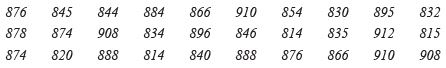Construct a frequency distribution table with class size 10.
Solution:
The lowest observation = 814
The highest observation = 912
Class size = 10
To cover the given data we have the classes as: 814–824, 824–834, …, 904–914.
Thus the required frequency distribution is as under: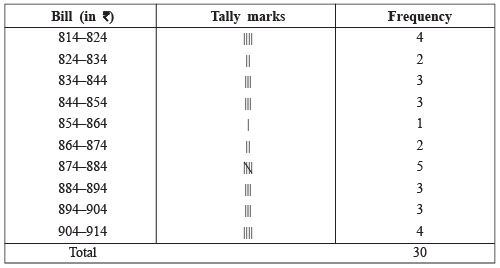Note: A frequency distribution in which each upper limit of each class is excluded and the lower limit is included, is called an exclusive form or the continuous form whereas on the other hand a frequency distribution in which each upper limit as well as lower limit is included, is called an inclusive form.

Question 3. Following data gives the marks (out of 50), obtained by 30 students of a class in a test: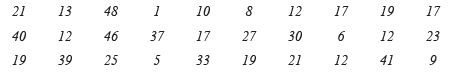Arrange the data using classes as 0–10, 11–20, etc.
Solution:
The lowest observation = 1
The highest observation = 48
∴ To cover the given data the required classes will be: 0–10, 11–20, …, 41–50.
Now, we have: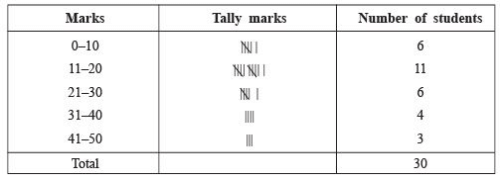Question 4. If the mean of the following data is 18.75, then find the value of p.

 xi 10 15 p 25 30 fi 5 10 7 8 2

Solution: Writing the given data as in the following table.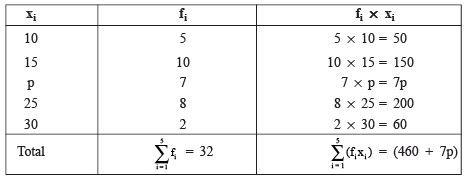∴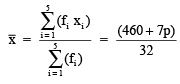But the mean of the given data is 18.75.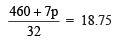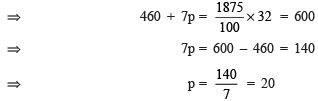Thus, the required value of p is 20.

Question 5. If the marks of 41 students of a class are given in the following table, then find the median of marks obtained.

 Marks obtained Frequency 30 25 27 40 32 35 10254128

Solution: Arranging the observations in ascending order, we have:

 Marks obtained 25 27 30 32 35 40 Frequency 2 5 10 12 8 4

Now, we prepare the cumulative frequency table as given below:

 Marks obtained Frequency Cumulative frequency 252730323540 25101284 2717293741

∵ Total number of observations = 41, (an odd number)

∴ Median of marks = marks obtained by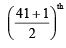student = marks obtained by the 21st student
∵ 18th to 29th students get 22 marks. i.e. 21st student gets 22 marks.
∴ The median of the marks = 22.

Question 6. Prove that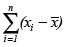= 0, where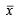is the mean of the ‘n’ observations x1, x2, x3, x4,
…, xn.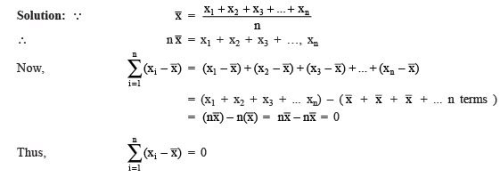The document Practice Questions: Statistics Notes | Study Mathematics (Maths) Class 9 - Class 9 is a part of the Class 9 Course Mathematics (Maths) Class 9.
All you need of Class 9 at this link: Class 9Use Code STAYHOME200 and get INR 200 additional OFF

## Mathematics (Maths) Class 9

73 videos|351 docs|110 tests

Track your progress, build streaks, highlight & save important lessons and more!

,

,

,

,

,

,

,

,

,

,

,

,

,

,

,

,

,

,

,

,

,

;